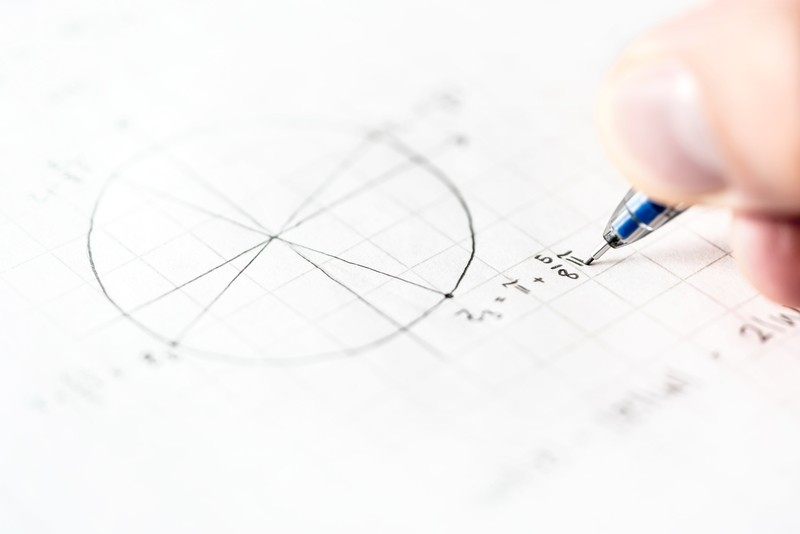# Math Skills That Come in Handy When Taking the GEDThe GED is a test that you can take if you do not have a high school diploma. While this test covers many subjects including science, language arts, and social studies, you may find that you’ll want to spend extra time studying for the math section. Math is commonly a trickier portion and there are some skills you should master before taking the test.

## Geometry

Geometry is a part of math that is often used in many other subject areas. You will likely be asked questions about the area, perimeter, volume, etc. All of these require that you understand the fundamentals of shapes and how to manipulate them. As you study, it may be helpful to use 3D shapes to better visualize and understand the shapes. You can also make flashcards to help you remember important concepts. If you understand the basics, you can apply them to more complex math problems.

## Data Analysis

Data analysis is a skill that can be applied to the real world. When using data analysis, you can look over a graph or data set and understand what it is trying to convey. Fortunately, this is a skill that can be used in many areas. You’ll need data analysis skills on the science portion of the GED, as well as on the math portion. On the test, you may be asked something as simple as interpreting a graph or you may be asked to make calculations. For example, you might calculate the mean, median, and mode. Do plenty of practice problems to get used to using this skill.

## Algebra

Algebra is a more complex type of math, but it too can be applied to many other areas. When you use algebra, you are mostly working with different types of equations and variables. You must understand basic math functions (addition, subtraction, multiplication, etc.) to understand algebra. It can be helpful to remember the acronym PEMDAS (parenthesis, exponents, multiplication, division, addition, subtraction) which tells you the order to do equations. The most important thing to remember though is to show your work. This keeps you from getting confused while working through equations. Studying and practicing problems will be beneficial in getting you comfortable with algebra.

Math is one of the most challenging subjects. To excel in the math portion of the GED, you must learn certain concepts and principles. These will help you to be better prepared.

Math Skills That Come in Handy When Taking the GED
Scroll to top